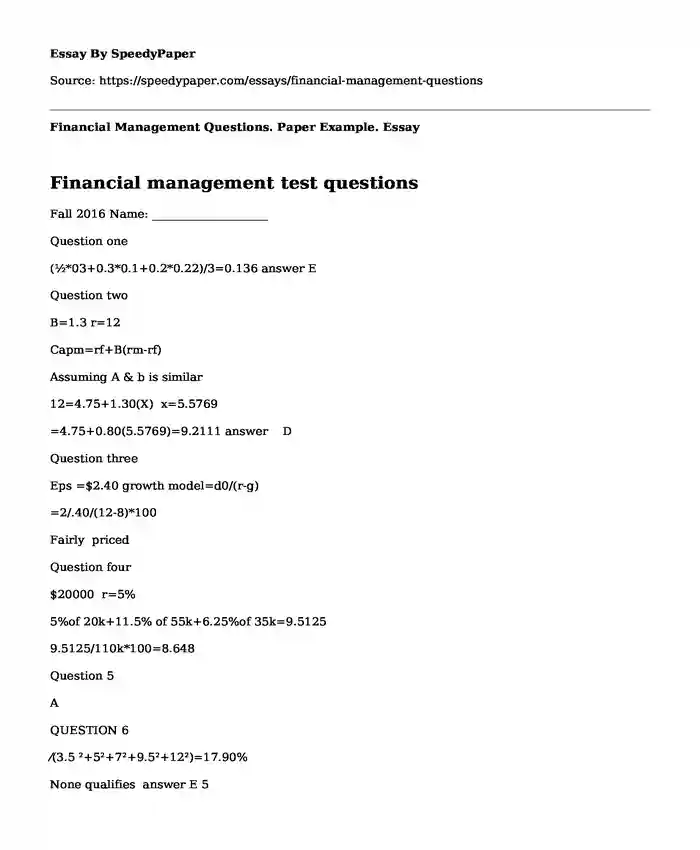# Financial Management Questions. Paper Example.

Published: 2017-12-18 03:49:16Type of paper: Essay Categories: Financial management Pages: 2 Wordcount: 465 words
143 views

## Financial management test questions

Fall 2016 Name: ____________________

Is your time best spent reading someone else’s essay? Get a 100% original essay FROM A CERTIFIED WRITER!

Question one

Question two

B=1.3 r=12

Capm=rf+B(rm-rf)

Assuming A & b is similar

12=4.75+1.30(X) x=5.5769

Question three

Eps =\$2.40 growth model=d0/(r-g)

=2/.40/(12-8)*100

Fairly priced

Question four

\$20000 r=5%

5%of 20k+11.5% of 55k+6.25%of 35k=9.5125

9.5125/110k*100=8.648

Question 5

A

QUESTION 6

⁄(3.5 ²+5²+7²+9.5²+12²)=17.90%

Question seven

Value of stock=dividend/r-g

\$35.59=1.25/r-7%

R=3.51%+7%=10.51%

10.51=4+b(6.75)

B=0.94

Question eight

Future value =p .v(1+R)n

75000(1+7.5) *30=

656621.6392

After tax =0.72*656621.63=472767 + discounted 401k =543,662.5

Question nine

Question ten

Discounting FCF

=p(1+r)^n

65=p(1+6.5%)05=47.442

Vo=do(1+g)n

47.442/14-6.5=6.3256/5=1.2

## Financial management exam questions and answers

Question 11

2.8/r-3.8=26.91

R=0.1040*100=10.40+3.8=14.21

Question 12

Question 13

Vo=do/r

R=5/35.79=12.59%

Question 14

2.4+1.35(6.8)

=11.

Question 15

Question 16

Question 17

Question 18

0.85*50k+100k*1+75k*1.25/3

Question 19

450 shares prices is 12.30

Question 20

Question 21

Income, age ,tax ,withdrawal ,rules

Q 23

Traditional IRA is a personal retirement account through which a person contributes pretax dollars and enables one’s money to increase tax deferred.

Roth IRA is where individuals pay taxes on all money that enters their account but has tax free future withdrawals. It is sensible to individuals who believe that their rate of taxation will be higher in retirement than their current state.

Question 24

Collective ,individual and group investments

Q 25

A short sale is the sale of real estate where net proceeds accruing from property sales are less than debts that are secured by liens against properties. The strategy behind short sales is to assist homeowners that may find themselves under water when they are not able to pay for taxes and mortgages. However, the risk is that short sales take too long with one risking losing all his money.

Question 26

It is reasonable for them to use 10% because Wacc is equitable to the rate of return The reason weight average cost of capital would be used as benchmark to analyze potential cash flow is because it is equal the required rate of return given it represent the opportunity cost in discounting growth model

The weight average cost of capital represent the opportunity cost put into use either as debt or equity by investors or owners.

Q 27

The primary reason a firm would choose to perform a stock split is to raise capital with the current shareholders and increase the numbers of shares to be able to trade in the stock exchange.

A firm would do a share split to fulfill the obligation of the regulator in meeting requirement for operating in the stock exchange.

It is also a way of consolidating large number of shares floating in the market.

A company would perform a reverse Stock split in order to realize an increment in its share prices.